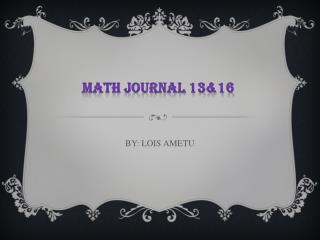DownloadDownload PresentationBY: LOIS AMETU

BY: LOIS AMETU

Download PresentationBY: LOIS AMETU

- - - - - - - - - - - - - - - - - - - - - - - - - - - E N D - - - - - - - - - - - - - - - - - - - - - - - - - - -
Presentation Transcript

1. Math journal 13&16 BY: LOIS AMETU

2. What is a solution? Can an equation have more than one solution? No solutions? How are solutions related to identities? Give at least 3 examples of each. A solution is a number or number s that make an equation true, when substituted for a variable. An equation can have more than one solution and it can also have no solution. Identities is when every number is a solution. Example 1: x=3 2:x=6 3:x=2 4:0x=0(more than one solution)

3. Describe how to solve a basic equation using the “cover-up” method. Give at least 3 examples. To solve an equation using the cover-up method 1: cover up all part of the equation involving the variable. 2: look at what is left and find the number that makes the equation true. 3: repeat if needed. Examples 1:2x+4=10 2: 3x+5=11 3: 5x+4=24 ----+4=10 -----+5=11 ------+4=24 6+4=10 6+5=11 20+4=24 2x=6 3x=6 5x=20 X=3 x=2 x=4

4. Describe how we maintain balance in an equation. Explain the parts of an equation and how they help us solve them. Give at least 3 examples. To maintain a balance in an equation you do the same thing to the left and right side. The parts of an equation are the right hand side ,the middle and the left hand side. It help us solve them because we will know where the equal sign is. Examples: 1: x-5=2 2: x-7=0 3: x+3=2 +5 +5 +7 +7 -3 -3 x-10=7 x-14=7 x+0=-1

5. Describe what an inverse operation is. What are some examples of inverse operations that we have already learned this year? Give at least 3 examples. Inverse operation is doing the opposite, example, +/-, */÷. Examples 1: -3=+3 2: +5=-5 3: *8=÷8 4: ÷12=*12

6. Describe how to solve one-step algebraic equations. Give at least 3 examples. To solve one step algebraic equation you look at what is being done to the x and do the inverse operation to both sides of the equation Examples 1: X+2=8 2: x-4=7 3: x+5=15 -2 -2 +4 +4 -5 -5 X=6 x=11 x=10

7. Describe how to “build up” and “undo” algebraic expressions. Give at least 3 examples of each. To build up algebraic equation you use the order of operation . To undo algebraic expression do the order of operation backwards with inverse operation. Example :build and undo 1: 4x+1 3: 7x-9 X(4)=4x+1=4x+1 x(7)=7x-9=7x-9 4x+1-1=4x÷4=x 7x-9+9=7÷7=x 2: 3x+8 X(3)=3x+8=3x+8 3x+8-8=3x÷3=x

8. Describe how to solve two-step and multi-step algebraic equations. Give at least 3 examples. To solve two step equation you. 1: undo +/-using inverse operation . 2: undo the */÷ using inverse operation.*undo the side with x. To solve multi step equation you undo the side with the x by doing inverse operation to both sides while doing the order of operation backwards. Example 1: 5(2x-6)=50 2x÷2=16÷2 2: 3x+4=17 3: 3x-7=14 5(2x-6)=50÷5 x=8 -4 -4 +7 +7 2x-6=10 3x ÷3=13÷3 3x÷3=21÷3 +6 +6 x=13 x=7 3

9. Describe how to solve number puzzles using inverse operations. Give at least 3 examples. To solve number puzzle using inverse operation you translate it and follow order of operation Example I think of a number and subtract 5 from it and get the answer of 16 X-5=16 x=21 I think of a number multiply it by 4 and then add 6. the result is 18 4n+6=18 n=3 I think of a number add 6 from it and get the answer 24 X +6=24 x=18

10. Describe how to solve equations with variables on both sides of the equation. Give at least 3 examples. 1: simplify both sides of the equation and combine like terms 2: look at which variable is smaller and get rid of it from both sides 3: solve like normal Example 1: 2x+3=x+8 X=5 2: 3x-1=7+x X=4 3: 4x+6=2x+6 X=0

11. Describe the 7-step process for solving any application problem. Give at least 3 examples. 1: read the problem carefully. 2:what is the question asking 3:draw a picture if possible label it with important information .4:translate to make an equation. 5: solve the equation. 6:answer the question . 7:check your answer does it make sense.

12. Describe how to use translation and substitution to solve equations with more than one variable. 1 : look for one variable that is equal to an expression. 2: substitute that variable in the other equation. 3:solve . 4: replace to solve the other variable. 5:check your solution.